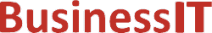O nás     Inzerce     KontaktSpolehlivé informace o IT již od roku 2011[Linux manuál]

# nextafter, nextafterf, nextafterl, nexttoward, nexttowardf, nexttowardl: další reprezentovatelné číslo s pohyblivou řádovou čárkou

Originální popis anglicky: nextafter, nextafterf, nextafterl, nexttoward, nexttowardf, nexttowardl - next representable floating-point number

Návod, kniha: POSIX Programmer's Manual

## STRUČNĚ

#include <math.h>

double nextafter(double x, double y);

float nextafterf(float x, float y );

long double nextafterl(long double x, long double y);

double nexttoward(double x, long double y );

float nexttowardf(float x, long double y );

long double nexttowardl(long double x, long double y);

## POPIS / INSTRUKCE

The nextafter(), nextafterf(), and nextafterl() functions shall compute the next representable floating-point value following x in the direction of y. Thus, if y is less than x, nextafter() shall return the largest representable floating-point number less than x. The nextafter(), nextafterf(), and nextafterl() functions shall return y if x equals y.
The nexttoward(), nexttowardf(), and nexttowardl() functions shall be equivalent to the corresponding nextafter() functions, except that the second parameter shall have type long double and the functions shall return y converted to the type of the function if x equals y.
An application wishing to check for error situations should set errno to zero and call feclearexcept(FE_ALL_EXCEPT) before calling these functions. On return, if errno is non-zero or fetestexcept(FE_INVALID | FE_DIVBYZERO | FE_OVERFLOW | FE_UNDERFLOW) is non-zero, an error has occurred.

## NÁVRATOVÁ HODNOTA

Upon successful completion, these functions shall return the next representable floating-point value following x in the direction of y.
If x== y, y (of the type x) shall be returned.
If x is finite and the correct function value would overflow, a range error shall occur and ±HUGE_VAL, ±HUGE_VALF, and ±HUGE_VALL (with the same sign as x) shall be returned as appropriate for the return type of the function.
If x or y is NaN, a NaN shall be returned.
If x!= y and the correct function value is subnormal, zero, or underflows, a range error shall occur, and either the correct function value (if representable) or 0.0 shall be returned.

## CHYBY / ERRORY

These functions shall fail if:
Range Error
The correct value overflows.
If the integer expression (math_errhandling & MATH_ERRNO) is non-zero, then errno shall be set to [ERANGE]. If the integer expression (math_errhandling & MATH_ERREXCEPT) is non-zero, then the overflow floating-point exception shall be raised.
Range Error
The correct value is subnormal or underflows.
If the integer expression (math_errhandling & MATH_ERRNO) is non-zero, then errno shall be set to [ERANGE]. If the integer expression (math_errhandling & MATH_ERREXCEPT) is non-zero, then the underflow floating-point exception shall be raised.

The following sections are informative.

None.

## APPLICATION USAGE

On error, the expressions (math_errhandling & MATH_ERRNO) and (math_errhandling & MATH_ERREXCEPT) are independent of each other, but at least one of them must be non-zero.

None.

None.

## SOUVISEJÍCÍ

feclearexcept() , fetestexcept() , the Base Definitions volume of IEEE Std 1003.1-2001, Section 4.18, Treatment of Error Conditions for Mathematical Functions, <math.h> Portions of this text are reprinted and reproduced in electronic form from IEEE Std 1003.1, 2003 Edition, Standard for Information Technology -- Portable Operating System Interface (POSIX), The Open Group Base Specifications Issue 6, Copyright (C) 2001-2003 by the Institute of Electrical and Electronics Engineers, Inc and The Open Group. In the event of any discrepancy between this version and the original IEEE and The Open Group Standard, the original IEEE and The Open Group Standard is the referee document. The original Standard can be obtained online at http://www.opengroup.org/unix/online.html .
 2003 IEEE/The Open Group
©2011-2020 BusinessIT.cz, ISSN 1805-0522 | Názvy použité v textech mohou být ochrannými známkami příslušných vlastníků.
Provozovatel: Bispiral, s.r.o., kontakt: BusinessIT(at)Bispiral.com | Inzerce: Best Online Media, s.r.o., zuzana@online-media.cz
O vydavateli | Pravidla webu BusinessIT.cz a ochrana soukromí | pg(9459)